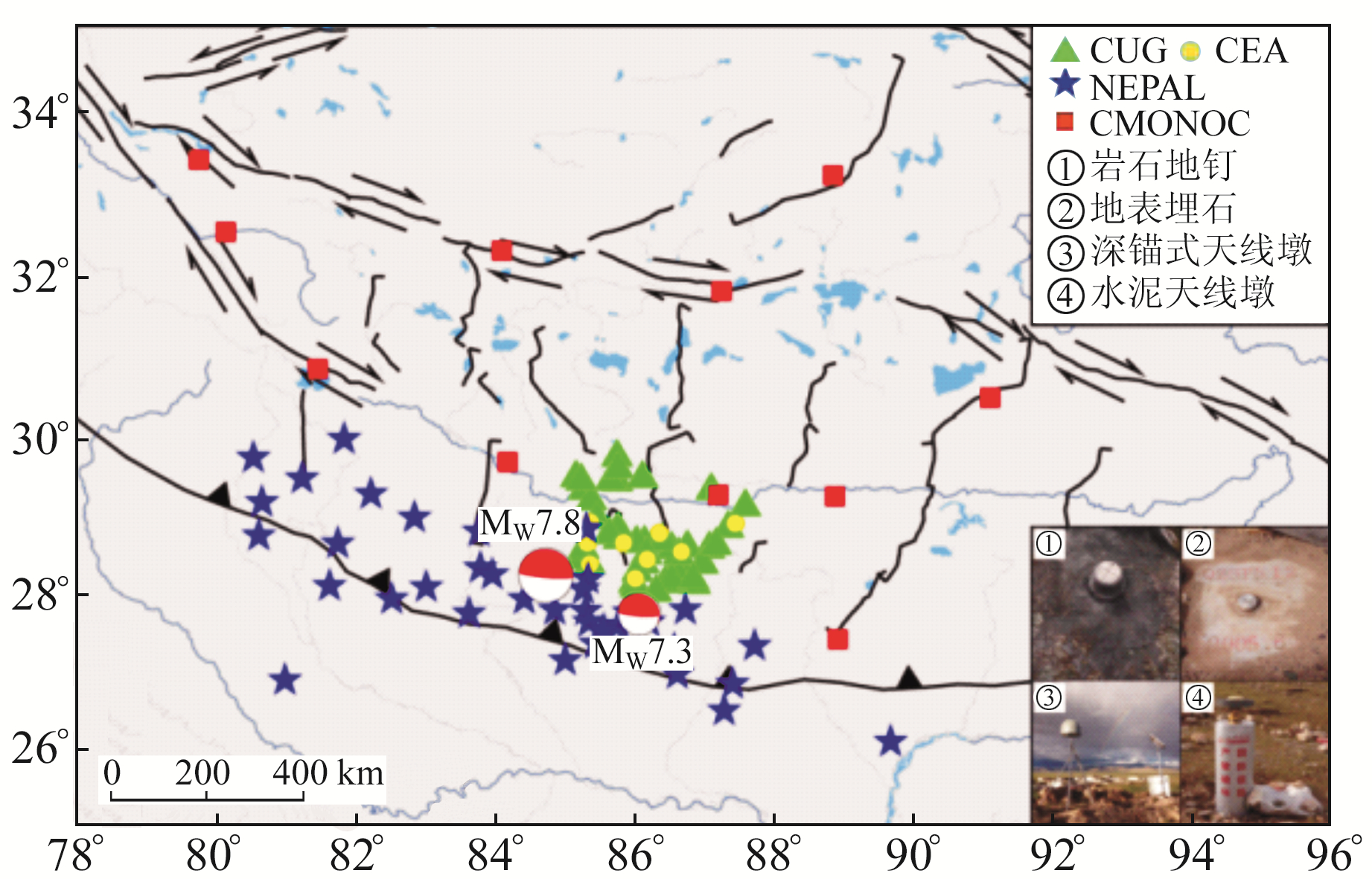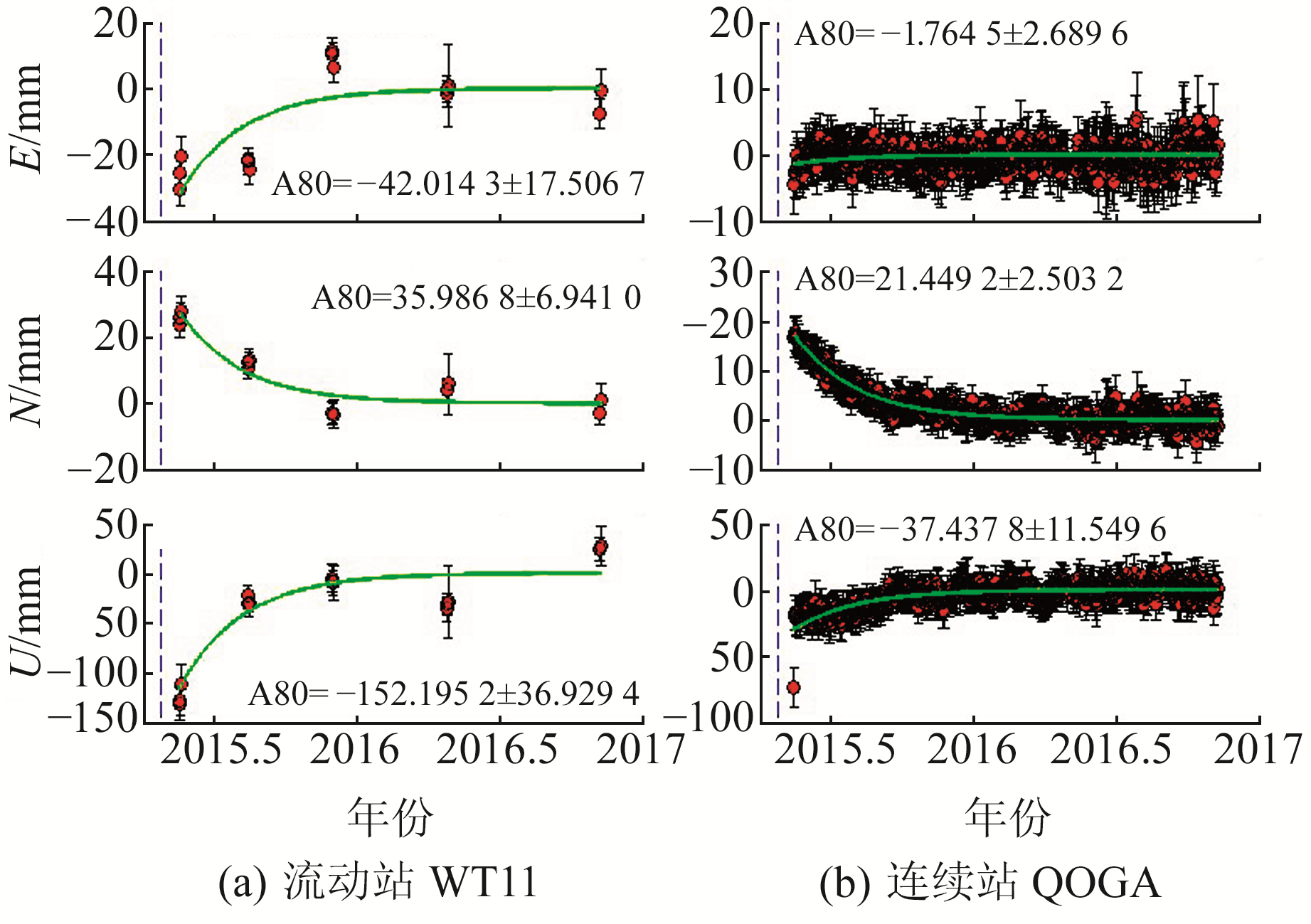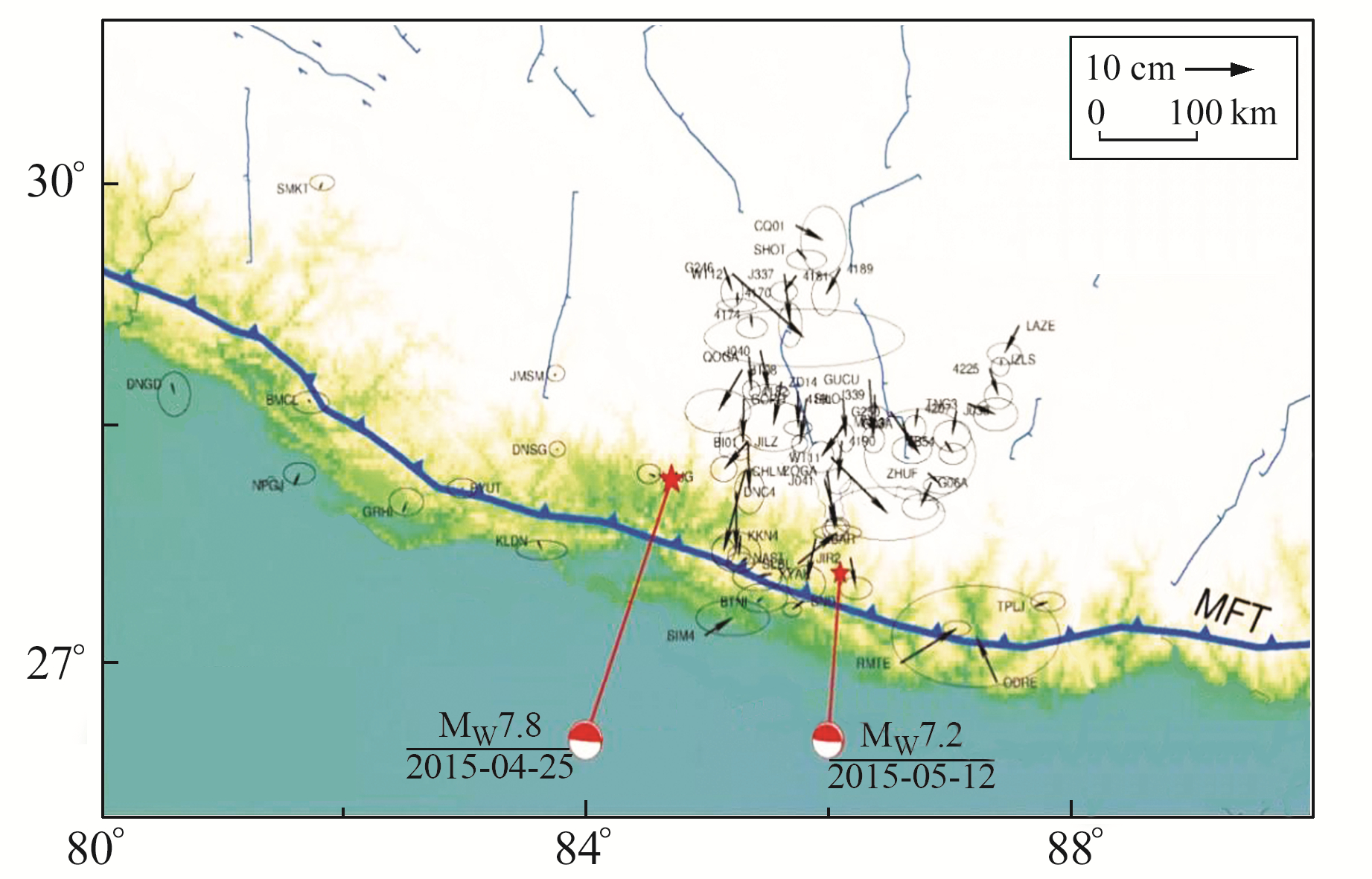﻿ 2015年尼泊尔M<sub>W</sub>7.8地震近场GNSS时间序列分析文章快速检索 高级检索
 大地测量与地球动力学2023, Vol. 43Issue (4): 380-386  DOI: 10.14075/j.jgg.2023.04.009### 引用本文WANG Li. Analysis of Near Field GNSS Time Series of the MW7.8 Earthquake in Nepal[J]. Journal of Geodesy and Geodynamics, 2023, 43(4): 380-386.### Foundation support

National Natural Science Foundation of China, No. 41674017, 41731071.

### 第一作者简介

WANG Li, engineer, majors in surveying and mapping, E-mail: wanglicug@126.com.

### 文章历史

2015年尼泊尔MW7.8地震近场GNSS时间序列分析
1. 惠州大亚湾经济技术开发区测绘产品质量检测中心，广东省惠州市北澳大道2号，516081

1 数据收集与解算表 1 GNSS测站统计 Tab. 1 Statistics of GNSS station图 1 GNSS测站分布 Fig. 1 GNSS station distribution

2 原理与方法 2.1 时间序列模型拟合

GNSS测站观测的时间序列可用如下线性多项式来表示

 $\begin{gathered} y\left(t_i\right)=a+b t_i+c \sin \left(2 \pi t_i\right)+d \cos \left(2 \pi t_i\right)+ \\ e \sin \left(4 \pi t_i\right)+f \cos \left(4 \pi t_i\right)+ \\ \sum\limits_{j=1}^{n_h} g_j H\left(t_i-T_{\mathrm{eq}}\right)+\sum\limits_{j=1}^{n_j} h_j H\left(t_i-T_{\mathrm{post}}\right) t_i+ \\ \sum\limits_{j=1}^{n_g} k_j \exp \left(-\left(t-T_{\mathrm{post}}\right) / \tau_j\right) H\left(t_i-T_{\mathrm{post}}\right)+v_i \end{gathered}$ (1)

2.2 周期性分析

GNSS时间序列可以展现地表在时间变化下的特性，如因板块运动的线性效应及周期性效应等。在周期性效应中，最为常见的为年周期与半年周期，该效应一般被认为由3种原因造成：1)由日、月潮引力造成的影响; 2)由太阳为热源造成的一系列周期变化，如大气压力、非潮汐所引起的海平面变化、地下水效应及热膨胀效应、季节性的降雨等; 3)由GNSS解算模型改正误差引起的周期性变化，如多路径效应、天线相位中心改正等。Dong等分析全球429个GPS连续站周期性效应显示，水平方向的年周期振幅范围在1~3 mm，高程方向则在4~10 mm。

 \left\{\begin{aligned} \Delta e= & \frac{R}{\sin \theta} \sum\limits_{n=1}^{\infty} \sum\limits_{m=0}^n \bar{P}_{n m}(\cos \theta) m\left[-\Delta \bar{C}_{n m} \cdot\right. \\ & \left.\sin (m \varphi)+\Delta \bar{S}_{n m} \cos (m \varphi)\right] \frac{l^{\prime}}{1+k^{\prime}}{ }_n \\ \Delta n= & -R \sum\limits_{n=1}^{\infty} \sum\limits_{m=0}^n \frac{\partial}{\partial \theta} \bar{P}_{n m}(\cos \theta)\left[\Delta \bar{C}_{n m} \cdot\right. \\ & \left.\cos (m \varphi)+\Delta \bar{S}_{n m} \sin (m \varphi)\right] \frac{l_n^{\prime}}{1+k_n^{\prime}} \\ \Delta h= & R \sum\limits_{n=1}^{\infty} \sum\limits_{m=0}^n \bar{P}_{n m}(\cos \theta) \cdot\left[\Delta \bar{C}_{n m} \cdot \cos (m \varphi)+\right. \\ & \left.\Delta \bar{S}_{n m} \cdot \sin (m \varphi)\right] \cdot \frac{h^{\prime}{ }_n}{1+k^{\prime}} \end{aligned}\right. (2)表 2 不同噪声模型估计速度、同震和震后结果 Tab. 2 Estimated velocity, coseismic and post-seismic results of different noise models图 7 震后位移时间序列 Fig. 7 Post-earthquake displacement time series
3.4 同震和震后位移图 8 尼泊尔MW7.8地震同震位移场 Fig. 8 Coseismic horizontal displacement field of MW7.8 Nepal earthquake图 9 尼泊尔地震GNSS观测震后20个月水平位移场 Fig. 9 Horizontal displacement field 20 months afterthe Nepal earthquake by GNSS observation
4 结语

1) GRACE改正GNSS数据，使得时间序列3个分量的WRMS平均降低15.52%、26.41%和45.06%;

2) 采用存在缺失数据的PCA方法获得第一主成分并计算共模误差，3个分量有效率达到61.91%、53.91%和53.88%;

3) 频谱分析确定WN+FN为最佳噪声模型，新的噪声模型得出的震间速度、同震和位移中误差是常用的WN模型结果的约6~9倍、4~7倍和2~4倍，即采用WN模型得出的中误差低估了真实值。

4) 通过对尼泊尔地震近场GNSS站点时间序列进行分析可知，结合Mencin等提供的震后余滑模型，尼泊尔地震震后形变主要发生在北部区域，且最大震后位移形变可达约60 mm。

  姜卫平, 王锴华, 李昭, 等. GNSS坐标时间序列分析理论与方法及展望[J]. 武汉大学学报: 信息科学版, 2018, 43(12): 2 112-2 123 (Jiang Weiping, Wang Kaihua, Li Zhao, et al. Prospect and Theory of GNSS Coordinate Time Series Analysis[J]. Geomatics and Information Science of Wuhan University, 2018, 43(12): 2 112-2 123) (0)  Nikolaidis R. Observation of Geodetic and Seismic Deformation with the Global Positioning System[D]. San Diego: University of California, 2002 (0)  Dong D, Fang P, Bock Y, et al. Anatomy of Apparent Seasonal Variations from GPS-Derived Site Position Time Series[J]. Journal of Geophysical Research: Solid Earth, 2002, 107(B4) (0)  Swenson S, Wahr J. Methods for Inferring Regional Surface-Mass Anomalies from Gravity Recovery and Climate Experiment(GRACE) Measurements of Time-Variable Gravity[J]. Journal of Geophysical Research: Solid Earth, 2002, 107(B9) (0)  Zou R, Wang Q, Freymueller J, et al. Seasonal Hydrological Loading in Southern Tibet Detected by Joint Analysis of GPS and GRACE[J]. Sensors, 2015, 15(12): 30 525-30 538 DOI:10.3390/s151229815 (0)  陈超, 邹蓉, 刘任莉. 联合GPS和GRACE研究青藏高原南部地区垂直形变的季节性波动[J]. 武汉大学学报: 信息科学版, 2018, 43(5): 669-675 (Chen Chao, Zou Rong, Liu Renli. Vertical Deformation of Seasonal Hydrological Loading in Southern Tibet Detected by Joint Analysis of GPS and GRACE[J]. Geomatics and Information Science of Wuhan University, 2018, 43(5): 669-675) (0)  Dong D, Fang P, Bock Y, et al. Spatiotemporal Filtering Using Principal Component Analysis and Karhunen-Loeve Expansion Approaches for Regional GPS Network Analysis[J]. Journal of Geophysical Research: Solid Earth, 2006, 111(B3): 1 581-1 600 (0)  Shen Y Z, Li W W, Xu G C, et al. Spatiotemporal Filtering of Regional GNSS Network's Position Time Series with Missing Data Using Principle Component Analysis[J]. Journal of Geodesy, 2014, 88(1): 1-12 DOI:10.1007/s00190-013-0663-y (0)  Mao A L, Harrison C G A, Dixon T H. Noise in GPS Coordinate Time Series[J]. Journal of Geophysical Research: Solid Earth, 1999, 104(B2): 2 797-2 816 DOI:10.1029/1998JB900033 (0)  赵斌, 杜瑞林, 张锐, 等. GPS测定的尼泊尔MW7.9和MW7.3级地震同震形变场[J]. 科学通报, 2015, 60(增2): 2 758-2 766 (Zhao Bin, Du Ruilin, Zhang Rui, et al. Co-Seismic Displacements Associated with the 2015 Nepal MW7.9 Earthquake and MW7.3 Aftershock Constrained by Global Positioning System Measurements[J]. Chinese Science Bulletin, 2015, 60(S2): 2 758-2 766) (0)  Mencin D, Bendick R, Upreti B N, et al. Himalayan Strain Reservoir Inferred from Limited Afterslip Following the Gorkha Earthquake[J]. Nature Geoscience, 2016, 9(7): 533-537 DOI:10.1038/ngeo2734 (0)
Analysis of Near Field GNSS Time Series of the MW7.8 Earthquake in Nepal
1. Surveying and Mapping Product Quality Testing Center of Huizhou Daya Bay Economic and Technological Development Zone, 2 Bei'ao Road, Huizhou 516081
Abstract: We collect the data of near-field intensive GNSS stations in Nepal, and use GAMIT/GLOBK software to calculate the data of 58 near-field continuous GNSS stations and 41 post-earthquake mobile GNSS stations in Nepal from 2011 to 2017. We analyze and process the periodicity, common mode error and noise of GNSS time series. The results show that: 1) The average WRMS of E, N and U components decreased by 15.52%, 26.41% and 45.06% after GRACE corrected the period term of GNSS time series; 2) In view of the large number of missing data in the time series, the traditional PCA method is improved to calculate the common mode error by using the first principal component, and the efficiency of the three components reached 61.91%, 53.91% and 53.88%, respectively; 3) Noise analysis shows that the optimal noise model of the Nepal earthquake near field GNSS station is WN+FN; compared with the WN model alone, the WN+FN model underestimates interseismic velocity, coseismic displacement and post-seismic displacement by about 6 to 9 times, 4 to 7 times and 2 to 4 times, respectively. The results of this paper are of great significance for the maintenance of regional coordinate frame, and the inversion of geophysical model of co-seismic rupture and post-earthquake residual slip of Nepal earthquake.
Key words: Nepal earthquake; near field GNSS; time series analysis; coseismic and post-earthquake